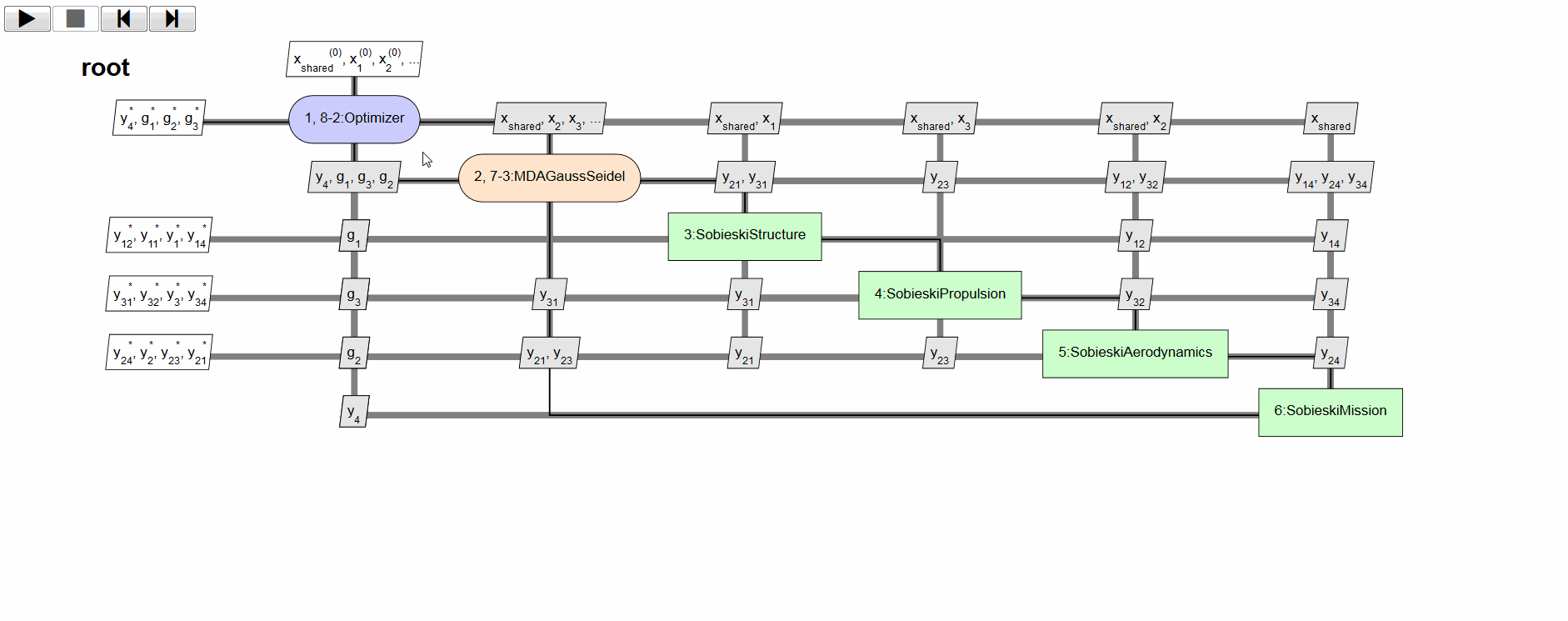# MDO formulations¶

In this section we describe the MDO formulations features of GEMSEO.

## Available formulations in GEMSEO¶

To see which formulations are available in your GEMSEO version, you may have a look in the folder gemseo.formulations. Another possibility is to use the API method gemseo.api.get_available_formulations() to list them:

from gemseo.api import get_available_formulations
print(get_available_formulations())


This prints the formulations names available in the current configuration.

['MDF', 'DisciplinaryOpt', 'BiLevel', 'IDF']

These implement the classical formulations:

In the following, general concepts about the formulations are given. The MDF and IDF text is integrally taken from the paper [VGM17].

To see how to setup practical test cases with such formulations, please see Tutorial: How to solve an MDO problem and sobieski_mdo.

For a review of MDO formulations, see [ML13].

We use the following notations:

• $$N$$ is the number of disciplines,

• $$x=(x_1,x_2,\ldots,x_N)$$ are the local design variables,

• $$z$$ are the shared design variables,

• $$y=(y_1,y_2,\ldots,y_N)$$ are the coupling variables,

• $$f$$ is the objective,

• $$g$$ are the constraints.

## MDF¶

MDF is an architecture that guarantees an equilibrium between all disciplines at each iterate $$(x, z)$$ of the optimization process. Consequently, should the optimization process be prematurely interrupted, the best known solution has a physical meaning. MDF generates the smallest possible optimization problem, in which the coupling variables are removed from the set of optimization variables and the residuals removed from the set of constraints:

\begin{split}\begin{aligned} & \underset{x,z}{\text{min}} & & f(x, z, y(x, z)) \\ & \text{subject to} & & g(x, z, y(x, z)) \le 0 \end{aligned} \label{eq:mdf-problem}\end{split}

The coupling variables $$y(x, z)$$ are computed at equilibrium via an MDA. It amounts to solving a system of (possibly nonlinear) equations using fixed-point methods (Gauss-Seidel, Jacobi) or root-finding methods (Newton-Raphson, quasi-Newton). A prerequisite for invoking is the existence of an equilibrium for any values of the design variables $$(x, z)$$ encountered during the optimization process.

Gradient-based optimization algorithms require the computation of the total derivatives of $$\phi(x, z, y(x, z))$$, where $$\phi \in \{f, g\}$$ and $$v \in \{x, z\}$$.

For details on the MDAs and coupled derivatives, see Multi Disciplinary Analyses and Coupled derivatives computation.

An example of an MDO study using an MDF formulation can be found in the Sellar MDO tutorial

## IDF¶

IDF handles the disciplines in a decoupled fashion: all disciplinary analysis are performed independently and possibly in parallel. Coupling variables $$y^t$$ (called targets) are driven by the optimization algorithm and are inputs of all disciplinary analyses $$y_i(x_i, z, y_{j \neq i}^t), \forall i \in \{1, \ldots, N\}$$. In comparison, handles the disciplines in a coupled manner: the inputs of the disciplines are outputs of the other disciplines.

\begin{split}\begin{aligned} & \underset{x,z,y^t}{\text{min}} & & f(x, z, y^t) \\ & \text{subject to} & & g(x, z, y^t) \le 0 \\ & & & y_i(x_i, z, y^t_{j \neq i}) - y_i^t = 0, \quad \forall i \in \{1, \ldots, N\} \end{aligned} \label{eq:idf-problem}\end{split}

Additional consistency constraints $$y_i(x_i, z, y^t_{j \neq i}) - y_i^t = 0, \forall i \in \{1, \ldots, N\}$$ ensure that the couplings computed by the disciplinary analysis coincide with the corresponding inputs $$y^t$$ of the other disciplines. This guarantees an equilibrium between all disciplines at convergence.

## Bi level¶

Bi level formulations are a family of MDO formulations that involve multiple optimization problems to be solved to obtain the solution of the MDO problem.

In many of them, and in particular in the formulations derived from BLISS, the separation of the optimization problems is made on the design variables. The shared design variables by multiple disciplines are put in a so called system level optimization problem. In so-called disciplinary optimization problems, only the design variables that have a direct impact on one discipline are used. Then, the coupling variables may be solved by a Multi Disciplinary Analyses, as in BLISS, ASO and CSSO, or by using consistency constraints or a penalty function, like in CO or ATC.

The next figure shows the decomposition of the bi-level MDO formulation implemented in GEMSEO MDAs, sub optimization and a main optimization on the shared variables. It is derived from the BLISS formulation and variants from ONERA [BILD12]. This formulation was invented in the MDA-MDO project at IRT Saint Exupery [GGG+17], [GGA+19].

## XDSM visualization¶

GEMSEO allows to visualize a given MDO scenario/formulation as an XDSM diagram (see [LM12]) in a web browser. The figure below shows an example of such visualization.

The rendering is handled by the visualization library XDSMjs. GEMSEO provides a utility class XDSMizer to export the given MDO scenario as a suitable input json file for this visualization library.

### Features¶

XDSM visualization shows:

• dataflow between disciplines (connections between disciplines as list of variables)

• optimization problem display (click on optimizer box)

• workflow animation (top-left contol buttons trigger either automatic or step-by-step mode)

Those features are illustrated by the animated gif below.GEMSEO XDSM visualization of the Sobiesky example solved with MDF formulation¶

### Installation¶

From GEMSEO v1.4, the manual installation of XDSMjs is not required, since a Python package is now available. Also, a self contained web page can be generated.

### Usage¶

Then within your Python script, given your scenario object, you can generate the XDSM json file with the following code:

scenario.xdsmize(open_browser=True)


If html_output (default True), will generate a self contained html file, that can be automatically open using the option open_browser=True. If outdir is set to Non (default ‘.’), a temporary file is generated. If json_output is True, it will generate a XDSMjs input file XDSM visualization (legacy behavior). If latex_output is set to True (default False), a Latex PDF is generated.

You should observe the XDSM diagram related to your MDO scenario.